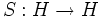# Hopf algebra

## History

### Motivation

The idea behind Hopf algebras was to generalize certain properties of the inverse map in the group algebra (which is the map that sends the basis elements to their inverses in the group and is extended linearly). It is/was also hoped that many properties that we study for groups and their group algebras, could also be studied for Hopf algebras.

## Definition

A Hopf algebra over a field is defined as a bialgebra over that field with an additional operation, called the antipode map, satisfying certain compatibility conditions.

### Notation

• Let$H$ be the set and$K$ the base field
• Let$\nabla$ denote the multiplication and$\eta$ the unit
• Let$\Delta$ denote the comultiplication and$\epsilon$ the counit
• Let$S:H \to H$ be a linear map which we call the antipode map

### Compatibility conditions

We require that$H$ equipped with the given multiplication, unit, comultiplication and counit forms a bialgebra, and that the following compatibility conditions hold:$\nabla \circ (S \otimes id) \circ \Delta = \nabla \circ (id \otimes S) \circ \Delta = \eta \circ \epsilon$

## Properties

Check out Category:Properties of Hopf algebras